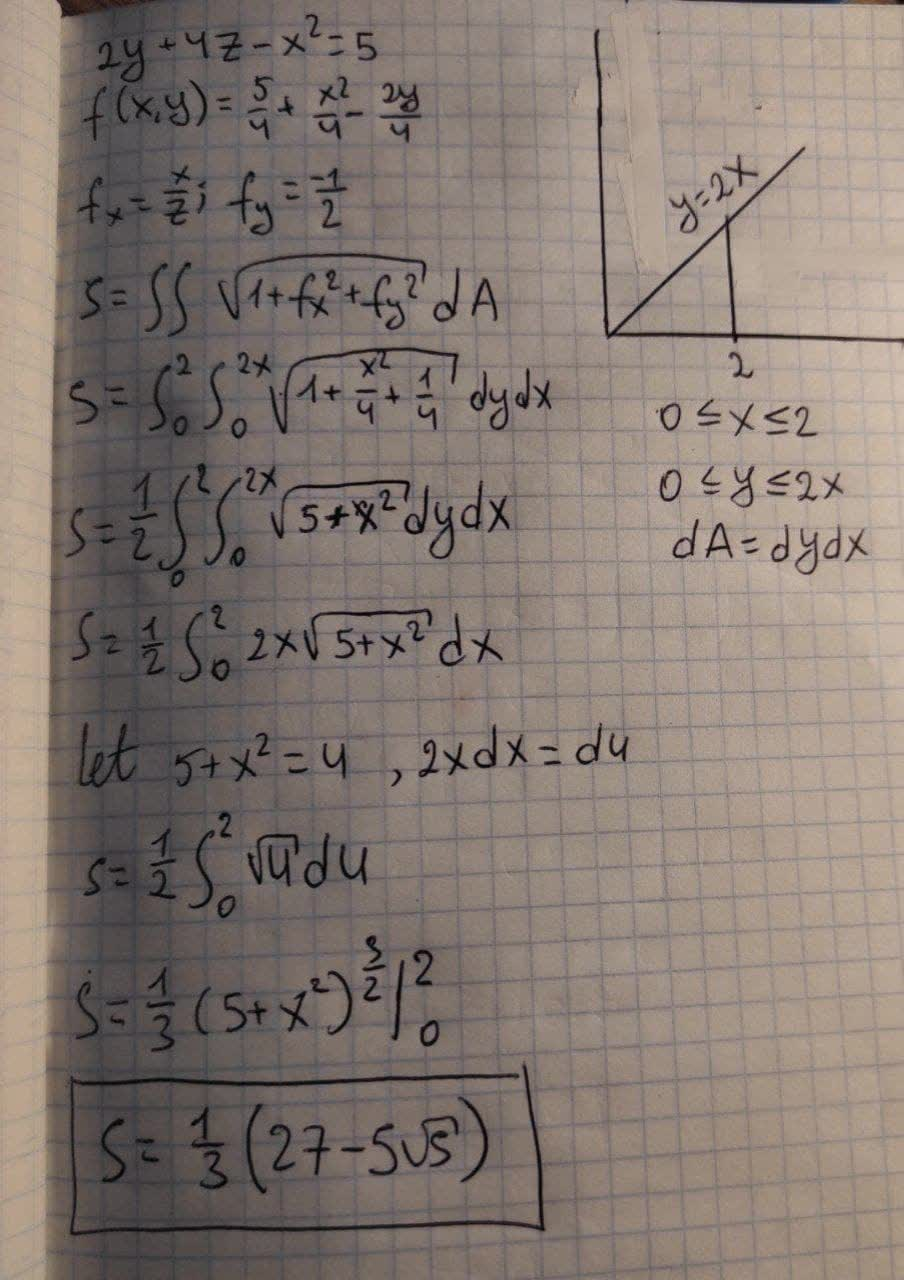Find the area of the surface. The part of theslaggingV 2021-08-15 Answered

Find the area of the surface. The part of the surface
$$2y + 4z − x^2 = 5$$
that lies above the triangle with vertices (0, 0), (2, 0), and (2, 4)

• Questions are typically answered in as fast as 30 minutes

Solve your problem for the price of one coffee

• Math expert for every subject
• Pay only if we can solve itopsadnojD

To find the area of the surface check photo below